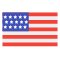enStyles
Categories
Trends
Old Interface
CollectionsView All
Drag icons
here

# Equal Icons

Approximately Equal
Collection
Approximately Equal
Collection
Approximately Equal
Collection
Approximately Equal
Collection
Approximately Equal
Collection
Approximately Equal
Collection
Not Equal
Collection
Not Equal
Collection
Not Equal
Collection
Not Equal
Collection
Not Equal
Collection
Not Equal
Collection
Not Equal
Collection
Not Equal
Collection
Not Equal
Collection
Not Equal
Collection
Not Equal
Collection
Not Equal
Collection
Not Equal
Collection
Not Equal
Collection
Not Equal
Collection
Not Equal
Collection
Not Equal
Collection
Not Equal
Collection
Not Equal
Collection
Not Equal
Collection
Not Equal
Collection
More or Equal
Collection
More or Equal
Collection
More or Equal
Collection
More or Equal
Collection
More or Equal
Collection
More or Equal
Collection
More or Equal
Collection
More or Equal
Collection
More or Equal
Collection
More or Equal
Collection
More or Equal
Collection
More or Equal
Collection
More or Equal
Collection
More or Equal
Collection
More or Equal
Collection
More or Equal
Collection
More or Equal
Collection
More or Equal
Collection
More or Equal
Collection
Less or Equal
Collection
Less or Equal
Collection
Less or Equal
Collection
Less or Equal
Collection
Less or Equal
Collection
Less or Equal
Collection
Less or Equal
Collection
Less or Equal
Collection
Less or Equal
Collection
Less or Equal
Collection
Less or Equal
Collection
Less or Equal
Collection
Less or Equal
Collection
Less or Equal
Collection
Less or Equal
Collection
Less or Equal
Collection
Less or Equal
Collection
Less or Equal
Collection
Less or Equal
Collection
Less or Equal
Collection
Less or Equal
Collection
Less or Equal
Collection
Less or Equal
Collection
Less or Equal
Collection
Less or Equal
Collection
Less or Equal
Collection
Less or Equal
Collection
Less or Equal
Collection
Less or Equal
Collection
Less or Equal
Collection
Less or Equal
Collection
Less or Equal
Collection
Less or Equal
Collection
Less or Equal
Collection
Less or Equal
Collection
Less or Equal
Collection
Less or Equal
Collection
Approximately Not Equal
Collection
Approximately Not Equal
Collection
Approximately Not Equal
Collection
Approximately Not Equal
Collection
Approximately Not Equal
Collection
Approximately Not Equal
Collection
Approximately Not Equal
Collection
Approximately Not Equal
Collection
Approximately Not Equal
Collection
Approximately Not Equal
Collection
Approximately Not Equal
Collection
Approximately Not Equal
Collection
Approximately Not Equal
Collection
Approximately Not Equal
Collection
Approximately Not Equal
Collection
Approximately Not Equal
Collection
Approximately Not Equal
Collection
Approximately Not Equal
Collection
Approximately Not Equal
Collection
Approximately Not Equal
Collection
Approximately Not Equal
Collection
Approximately Not Equal
Collection
Approximately Not Equal
Collection
Approximately Not Equal
Collection
Approximately Not Equal
Collection
Approximately Not Equal
Collection
Approximately Not Equal
Collection
Approximately Not Equal
Collection
Approximately Not Equal
Collection
Approximately Not Equal
Collection
Approximately Not Equal
Collection
Approximately Not Equal
Collection
Approximately Not Equal
Collection
Equality
Collection
Equality
Collection
Equality
Collection
Equals
Collection
Collections
0 set
Create new
Drag icons here